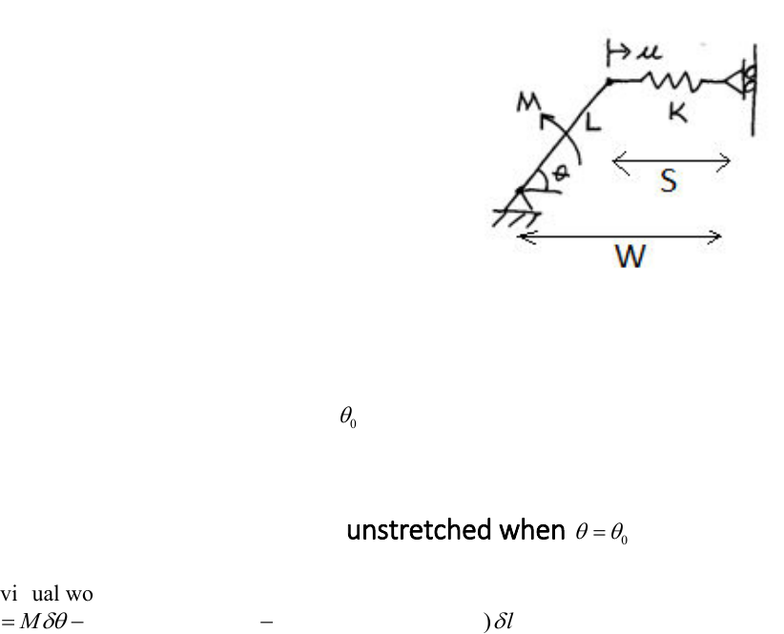Study Guides (390,000)
US (220,000)
TAMU (3,000)
AERO (10)
Final

# AERO 306 Lecture 4: 4_intro_instabilityExam

Department
Aerospace Engineering
Course Code
AERO 306
Professor
John Whitcomb
Study Guide
Final

This preview shows page 1. to view the full 4 pages of the document.C:\Users\cloudconvert\server\files\119\172\7\5244e4e0-7049-40c6-8a3d-
e2fd698f2f53\d7a5d8ea5eb5c2ce265a7175e9b83c.docx p. 1 of 4
Brief Introduction to Instability (Details will be covered in Chapter 4)
The results in this section were generated with the worksheet intro_instability_export_2.mw.
An equilibrium state can be stable or unstable. If it is unstable, a small perturbation in the
buckling of beam columns in some detail. This problem illustrates some of the concepts that we
will use later.
Looking at moment vs theta we can extract the
tangential stiffness (for 1 dof problem this is the
slope of force vs deflection curve), which is the
ratio of the increment in force to the increment in
displacement. The tangential stiffness, which we
will define to be KT, reveals whether the equilibrium
state is stable. Zero or negative tangential stiffness
for a one dof problem implies lack of stability.
If there is more than one degree of freedom, the
sign of the determinant of the "stiffness matrix" will
indicate stability. We will go into much greater detail later in the semester.
As you might expect, the behavior of the mechanism depends on the initial stretch of the spring.
We will first solve this problem assuming that there is some value of theta where the spring is
unstretched. We will define this angle as
0
.Then, we will consider a more general configuration
that includes the possibility that the spring is deformed regardless of the value of theta.
Solution assuming that spring is unstretched when
0

=
The virtual work is
()
unstretched
VW M K lengthOfSpring lengthOfSpring lengthOfSpring
=
Although we do not know the length of the spring, we do know that
The length of the spring = unstretched length - u
.
Therefore, the stretch of the spring = -u.
Hence, our challenge is to obtain an expression for u in terms of theta. It is worth noting that the
virtual work for this linear spring is the same whether we use the stretch or the compression of